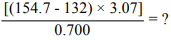# Problem: How many significant figures are there in the answer for the following problem?A) one B) two C) three D) four

###### FREE Expert Solution

We are asked for the number of significant figures in the answer to the problem.

The number of significant figures depends on the operation we use:

1.  addition or subtraction - the least number of decimal places will determine the final answer.

2. - the least number of significant figures will determine the final answer.

We operate the ones in the brackets first in this order : () → [] → {}

87% (210 ratings)###### Problem Details

How many significant figures are there in the answer for the following problem?A) one

B) two

C) three

D) four